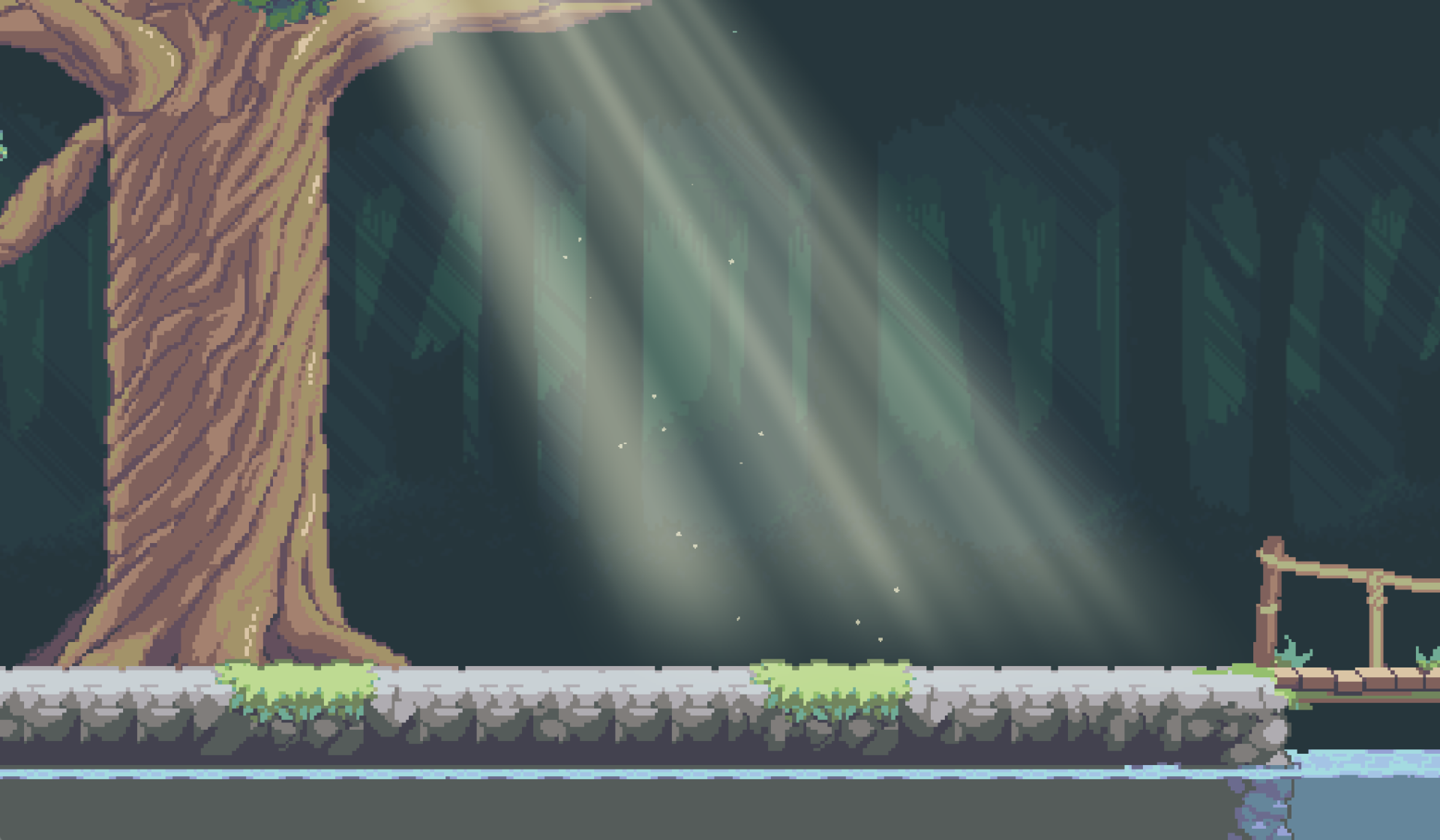# God rays

An animated god ray effect that can be used in many ways. See animation below.

Uniforms

• Angle – The angle of the rays.
• Position – Move the rays left and right.
• Cut off – The width of the effect.
• Fall off – Fade to transparent towards the bottom.
• Edge fade – The edges can be sharp or blurry.
• Speed – Speed of the “shimmer” animation.
• Ray 1 density – The effect has two layers of rays. This is how compact the back layer is, e.g how close together the gaps are. For best effect have the Ray 1 be wide and Ray 2 more compact.
• Ray 2 density – This is how compact the top layer is.
• Ray 2 intensity – Opacity of the top layer.

The rays blend with the background with a ‘Screen’ blending mode but you can change this for a different result. See godotshaders.com/snippet/blending-modes/ to get code to other blending modes.

Instructions
This shader is all code, no noise textures. So just paste the code into a new shader for a Sprite node or a ColorRect. Sprite nodes need a base texture.

``````/*

Feel free to use, improve and change this shader according to your needs
and consider sharing the modified result on godotshaders.com.
*/

uniform float angle = -0.3;
uniform float position = -0.2;
uniform float spread : hint_range(0.0, 1.0) = 0.5;
uniform float cutoff : hint_range(-1.0, 1.0) = 0.1;
uniform float falloff : hint_range(0.0, 1.0) = 0.2;
uniform float edge_fade : hint_range(0.0, 1.0) = 0.15;

uniform float speed = 1.0;
uniform float ray1_density = 8.0;
uniform float ray2_density = 30.0;
uniform float ray2_intensity : hint_range(0.0, 1.0) = 0.3;

uniform vec4 color : hint_color = vec4(1.0, 0.9, 0.65, 0.8);

uniform bool hdr = false;
uniform float seed = 5.0;

// Random and noise functions from Book of Shader's chapter on Noise.
float random(vec2 _uv) {
return fract(sin(dot(_uv.xy,
vec2(12.9898, 78.233))) *
43758.5453123);
}

float noise (in vec2 uv) {
vec2 i = floor(uv);
vec2 f = fract(uv);

// Four corners in 2D of a tile
float a = random(i);
float b = random(i + vec2(1.0, 0.0));
float c = random(i + vec2(0.0, 1.0));
float d = random(i + vec2(1.0, 1.0));

// Smooth Interpolation

// Cubic Hermine Curve. Same as SmoothStep()
vec2 u = f * f * (3.0-2.0 * f);

// Mix 4 coorners percentages
return mix(a, b, u.x) +
(c - a)* u.y * (1.0 - u.x) +
(d - b) * u.x * u.y;
}

mat2 rotate(float _angle){
return mat2(vec2(cos(_angle), -sin(_angle)),
vec2(sin(_angle), cos(_angle)));
}

vec4 screen(vec4 base, vec4 blend){
return 1.0 - (1.0 - base) * (1.0 - blend);
}

void fragment()
{

// Rotate, skew and move the UVs
vec2 transformed_uv = ( rotate(angle) * (UV - position) )  / ( (UV.y + spread) - (UV.y * spread) );

// Animate the ray according the the new transformed UVs
vec2 ray1 = vec2(transformed_uv.x * ray1_density + sin(TIME * 0.1 * speed) * (ray1_density * 0.2) + seed, 1.0);
vec2 ray2 = vec2(transformed_uv.x * ray2_density + sin(TIME * 0.2 * speed) * (ray1_density * 0.2) + seed, 1.0);

// Cut off the ray's edges
float cut = step(cutoff, transformed_uv.x) * step(cutoff, 1.0 - transformed_uv.x);
ray1 *= cut;
ray2 *= cut;

// Apply the noise pattern (i.e. create the rays)
float rays;

if (hdr){
// This is not really HDR, but check this to not clamp the two merged rays making
// their values go over 1.0. Can make for some nice effect
rays = noise(ray1) + (noise(ray2) * ray2_intensity);
}
else{
rays = clamp(noise(ray1) + (noise(ray2) * ray2_intensity), 0., 1.);
}

rays *= smoothstep(0.0, falloff, (1.0 - UV.y)); // Bottom
rays *= smoothstep(0.0 + cutoff, edge_fade + cutoff, transformed_uv.x); // Left
rays *= smoothstep(0.0 + cutoff, edge_fade + cutoff, 1.0 - transformed_uv.x); // Right

// Color to the rays
vec3 shine = vec3(rays) * color.rgb;

// Try different blending modes for a nicer effect. "Screen" is included in the code,
// but take a look at https://godotshaders.com/snippet/blending-modes/ for more.
// With "Screen" blend mode:
shine = screen(texture(SCREEN_TEXTURE, SCREEN_UV), vec4(color)).rgb;

COLOR = vec4(shine, rays * color.a);
}``````
###### Tags
effect, environment, light, shineThe shader code and all code snippets in this post are under CC0 license and can be used freely without the author's permission. Images and videos, and assets depicted in those, do not fall under this license. For more info, see our License terms.

## More from pend00

### VHS and CRT monitor effect

Subscribe
Notify ofInline Feedbacks2 years ago

it’s wonderful!!!!esa
2 years ago

Beautiful!agrogers
2 years agoLeonid
2 years ago

Fabulous effect !! I noticed that when exporting to android, only one ray is visible. Here’s a videoDeclivever
2 years agoJeno
11 months ago

is it possible to update this lovely shader to Godot 4?

‘hint’ seems to no longer be supported so the shader doesnt work in Godot 4Iliya
8 months ago

uniform sampler2D SCREEN_TEXTURE : hint_screen_texture, filter_linear_mipmap;

Replace hint_color to source_color

and all should work fine7 months agoNyaaa
5 months ago

If someone is using this shader in Godot 4 Vulkan and is getting weird streaks, change the random function to the following:

```float random(vec2 _uv) {
_uv += min(TIME,0.0).
return fract(sin(dot(_uv.xy.
vec2(12.9898, 78.233))) *
43758.5453123).
}
```RoseBloom
3 months ago